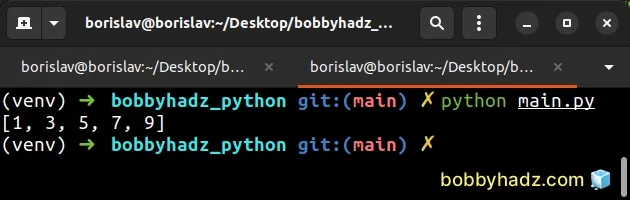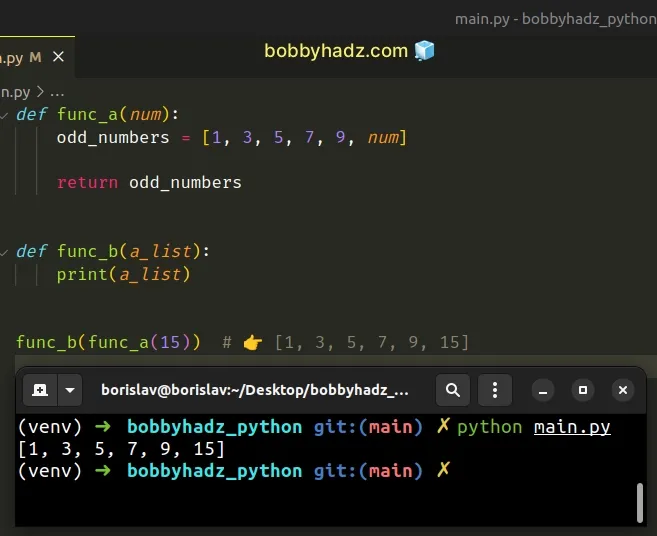# How to use a Variable from another Function in PythonLast updated: Jun 23, 2023
4 min## #How to use a Variable from another Function in Python

There are multiple ways that enable you to use a variable from another function in Python:

1. You can return the variable from the first function and store the return value in the second function.
2. The second function can take the variable as a parameter.
3. You could define the functions as class methods and access the variable on the `self` object.
4. You could use the `global` keyword to declare the variable as global.
5. You can also set function attributes that are accessible from other functions.

Let's look at an example of returning the variable from the first function.

main.py
```Copied!```def func_a():
odd_numbers = [1, 3, 5, 7, 9]

return odd_numbers

def func_b():
a_list = func_a()
print(a_list)

func_b()  # 👉️ [1, 3, 5, 7, 9]
``````The first function defines a variable and returns it using the `return` statement.

The second function invokes the first function using parentheses and stores its return value in a new variable.

If the functions take arguments, make sure to supply them as well.

main.py
```Copied!```def func_a(num):
odd_numbers = [1, 3, 5, 7, 9, num]

return odd_numbers

def func_b():
a_list = func_a(11)
print(a_list)

func_b()  # 👉️ [1, 3, 5, 7, 9, 11]
``````

The first function takes a number as an argument, so we had to pass it when calling it.

## #Passing the variable as an argument to the second function

Alternatively, you can pass the variable as an argument to the second function.

main.py
```Copied!```def func_a(num):
odd_numbers = [1, 3, 5, 7, 9, num]

return odd_numbers

def func_b(a_list):
print(a_list)

func_b(func_a(15))  # 👉️ [1, 3, 5, 7, 9, 15]
``````The first function returns a list of numbers using the `return` statement.

The second function takes a list as a parameter and prints it.

When calling the second function, we passed it the result of calling the first function.

The second function gets called with the list that is returned from the second function.

## #Wrapping the functions in a class

Alternatively, you can wrap the functions in a class.

main.py
```Copied!```class Employee():
def __init__(self, first, last):
self.first = first
self.last = last
self.salary = 100

def increase_salary(self):
self.salary += 100
print(self.salary)

def decrease_salary(self):
self.salary -= 100
print(self.salary)

emp1.increase_salary()  # 👉️ 200
emp1.increase_salary()  # 👉️ 300

emp1.decrease_salary()  # 👉️ 200
emp1.decrease_salary()  # 👉️ 100
``````

The `Employee()` class takes 2 parameters upon instantiation - `first` and `last`.

The `increase_salary` and `decrease_salary` methods don't take any parameters and can be called on a class instance.

The methods increase or decrease the `salary` instance variable.

You can optionally set a default value for the variable in the `__init__` method of the class.

The __init__() method is automatically run when the class is instantiated.

## #Using a Variable from another Function by marking it as global

You can also mark a variable as global to be able to use it in another function.

main.py
```Copied!```full_name = ''

def function_a():
global full_name

def function_b():
print(full_name)

function_a()
``````

We initialized the variable in the global scope, setting it to an empty string.

The `global` keyword enables us to update the value of the variable from the outer scope from within a function.

Once the variable's value has been updated, we can access the updated value in the other function.

Notice that we first called `function_a` to update the value of the global variable.

Had we called `function_b` first, the value of the variable would have been an empty string.

## #Use a variable from another function by setting function attributes

You can also use a variable from another function by setting function attributes.

main.py
```Copied!```def function_a():
print(function_a.website)

def function_b():
print(function_a.website)

``````

We used dot notation to set the `website` attribute in `function_a`.

You can access the attribute directly on the function object, e.g. `function_name.attribute_name`.

We are also able to access the attribute from other functions.

However, note that `function_a` has to be invoked first as the function sets the attribute upon invocation.

If you try to call `function_b` first, you would get an `AttributeError` exception because the attribute has not been set yet.